## Conventions

In case there's any confusion, the following computations belong to a setting where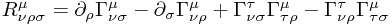and of course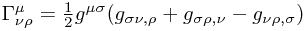## Warped Procucts

Suppose you have a space with coordinates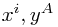of the form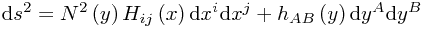so that schematically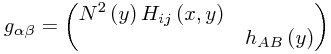This is a so-called warped product. A common question is "What is the form of the Ricci tensor?"

Let the dimension of the x subspace - that is to say, how many values i can range over - be denoted by D. Then one obtains the result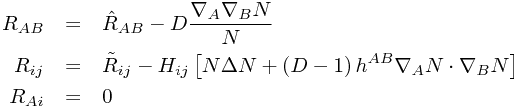where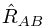is the Ricci tensor of the metric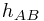andis the Ricci tensor of the metric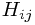. Note that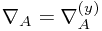is the covariant derivative with respect to themetric.

Observe that the form of the modification tois a multiple of the metric tensor for the x subspace.

## Conformal Rescalings

Another very common computation involves the rescaling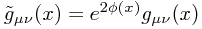A direct computation in local inertial coordinates for the new metric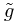gives the result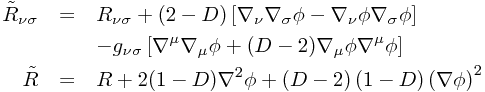where all covariant derivatives are measured in the unaltered metric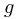, and all indices are raised and lowered using this metric too.